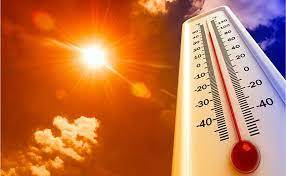TemperatureTemperature, a measure of hotness or coldness expressed in terms of several arbitrary scales and indicating the direction in which heat energy will spontaneously flow i.e. from hot body to cold body. Temperature is not the equivalent of the energy of a thermodynamic system; e.g., a burning match temperature is much higher than that of an iceberg but the total heat energy contained by the iceberg is much greater than the energy contained in a match. Temperature, similar to pressure or density, is called an intensive property    one that is independent of the quantity of matter being considered    as distinguished from extensive properties, such as mass or volume.

﻿Thermometer

A device that is used for measuring the temperature of a body is called a thermometer. A thermometer works on the basis of the principle "when a body is heated it expands and it contracts on cooling"

Thermometric liquid: A thermometric liquid is a liquid that can change its volume(increases or decreases) in accordance with the surrounding temperature. In other words, thermometric liquids are used in thermometers to measure temperature. The two types of thermometric liquids are:

1. Mercury: We all know that most metals are good conductors of heat, electricity and are solid at room temperature. Mercury is the only metal that is in a liquid state at room temperature. It is used in thermometers because it freezes at -39°C and boils at 357°C (which is a high coefficient of expansion) which makes it easy to move in the thermometers as per the temperature. It has a high boiling point so it is suitable to measure at higher temperatures.
2. Alcohol: Alcohol is also a thermometric liquid. The freezing point of alcohol is -117°C and the boiling point is 78°C. Thus, alcohol remains liquid to a very low temperature but not at a high temperature i.e. beyond 78°C. Due to this reason, an alcohol thermometer is used for measuring the temperature in very cold regions. The normal temperature of the human body is 27°C(98.6°F). The SI unit of temperature is Kelvin.

﻿Temperature scale

﻿General thermometers are calibrated in centigrade scale, Fahrenheit scale and Kelvin scale.

• Centigrade scale: In this scale, the lower and the upper fixed points are 0°C and 100°C respectively. The interval between these two fixed points is 100 equal parts. Each of the parts is called one-degree centigrade. It is denoted by 1°C.
• Fahrenheit scale: The lower and the upper fixed points are 32°F and 212°F respectively. The interval between these two points is divided into 180 equal divisions. Each one of the divisions is called one degree Fahrenheit. It is denoted by 1°F
• Kelvin scale: In the kelvin scale, the lower point is 273 K and the upper point is 373 K. The interval between these two points is divided into 100 equal divisions. Each of these divisions is called one kelvin. It is denoted by 1K.Heat Temperature The total kinetic energy of molecules that provide us with the sensation of warmth is called heat. The average kinetic energy of molecules in a substance that allows us to measure hotness or coldness is called temperature. SI unit is the joule. SI unit is Kelvin. Units are joules, Calories. Units are Fahrenheit, Celcius, Kelvin. Its symbol is Q. Its symbol is T. The calorimeter is used to measure it. The thermometer is used to measure it. It is caused by a change in temperature. It is the effect of heat.